# ILP Part 1 – Boolean algebra

If you are interested in the topic see the talk page

Today we are going to implement Boolean algebra in ILP!

# ILP – what’s that

Integer Linear Programming (ILP) is a way of formulating optimization problems. We define a set of variables, their upper and lower bounds, a set of constraints, and a cost function which we use to evaluate possible solutions. The main idea here is to use only linear functions (so we are not allowed to multiply variables), and require variables to be integers. If only some of the variables need to be integer, we have a Mixed Integer Linear Programming problem.

# Why bother with ILP

This branch of mathematics is widely used in allocation problems. If we need to optimize production in factories, then ILP is a good way to go. It is a very well explored concept and there are papers describing in detail how to solve ILP programs. There are also enterprise solvers like CPLEX or Gurobi, which are highly optimized and scalable. Considering that, it is relatively easy to solve an ILP problem, and because ILP is NP-Complete, we are able to use it to represent lots of problems (starting with sorting and finishing with Hamiltonian path).

# Let’s go

We are going to implement Boolean algebra in ILP. We are going to define constraints which will allow us to represent logical operators in ILP, so we will be able to create logical expressions.

## Notation

In examples we will use the following notation: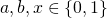We have three binary variables. We’ll use first two (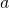and) as arguments for logical operator,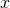will usually represent the result of the operation. Let’s go.

## Conjunction

We will start with conjunction. As you probably remember from school, it is defined in the following way: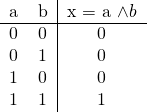Basically, the result is equal to one if and only if both arguments are equal to one. In ILP we can use the following formula: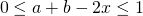These two inequalities fulfill the requirements. When bothandare equal to zero thencannot be equal to one (because the left inequality would not hold) and must be zero. When eitheroris equal to one the situation doesn’t change. However, if bothandare non-zero, thencannot be zero (because right inequality would not hold).

We can easily generalize this formula for multiple variables. Assuming thatare binary variables, we can do the following:You can conduct similar reasoning to proof that it is corrent.

## Disjunction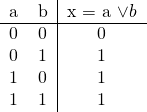Disjunction is very similar to conjunction. Basically, we need to swap bounds: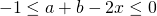Indeed, if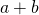is zero thenmust be zero too. However, if any ofandis equal to one thencannot be zero (because the right inequality would not be true) and it must be one. Generalization is simple.

## Other operators

Negation is easy as pie: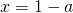To define implication we can use the identity:Exclusive or (XOR) goes this way:## More operators

You can implement other operators using identities or by stacking already defined ones. As an example try to define Sheffer stroke (also known as NAND gate).

# Summary

As we can see, implementing Boolean algebra is really straightforward and can be done without hacks or magical tricks. What’s more, solvers are aware of this construct and are able to recognize the logical relations (e.g., CPLEX has this ability) which probably leads to more efficient solving.
In the next part we are going to multiply variables in ILP.